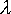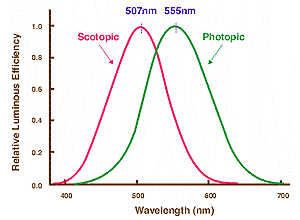QUESTION #409

# What is the equivalent of 1 Lux unit in Watts?

First, a technical point, Lux is a measure of illuminance, or luminous fLux per unit area, and so the corresponding quantity would be irradiance, or Watts per square meter. As for the answer, it depends on what color you're talking about. The Lux is a photometric unit, rather than a radiometric unit, which means that it takes into account the basic physics of energy and radiation, but also includes the physics of the human eye. Our eyes are simply better at picking up some colors than others. For instance, 1 Watt of yellow light would look a whole lot brighter (be absorbed more efficiently by the eye) than 1 Watt of blue light.

The conversion between photometric units (which take into account human physiology) and straight radiometric units is given by the following: (photometric unit) = (radiometric unit) x (685) x V(), where V() is the 'luminous efficiency,' and is a function of the wavelength of light. Basically the luminous efficiency tells us how efficiently the eye picks up certain wavelengths of light. Shown below is a graph of V() from the website given below in reference section.The photopic curve is for normal vision, and the scotopic curve is for eyes that are adjusted to the dark.

An interesting thing to note is that (for day vision) our eyes are most effective at picking up yellow light at around 555 nm - which is also the main wavelength of light emitted by our sun! This is evolution in action, our eyes evolved so as to most effectively utilize the resources available.

As for the question, working backwards from the equation given above will show that .0029 Watts of green light (= 510 nm, V() = 0.5) will provide an illuminance of 1 Lux, while only .0015 Watts of yellow light (= 555 nm, V() = 1) provide 1 Lux, and it takes a whopping .015 Watts of red light (= 650 nm, V() = 0.1) provide 1 Lux.

Reference: